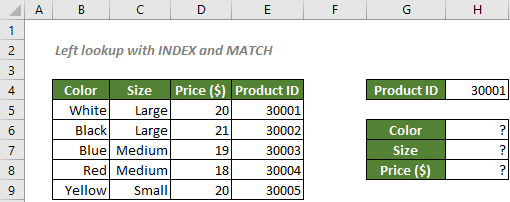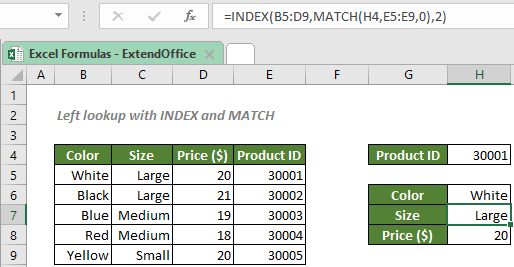## Left lookup with INDEX and MATCH

To find out information listed in the left columns in an Excel spreadsheet about a given item which is at the right side, you can use the INDEX and MATCH functions. The combination of the two functions possesses an advantage of looking up values in any column over another Excel’s powerful lookup function, the VLOOKUP.#### How to perform a left lookup with INDEX and MATCH?

To fill in the information about the product 30001 which is listed in the rightmost column of the data as shown in the screenshot above, the INDEX and MATCH functions will help you in this way: MATCH will find the correct row for the given product ID, and INDEX will then find the corresponding information on the same row.

#### Generic syntax

=INDEX(return_range,MATCH(lookup_value,lookup_array,[match_type]),column_num)

• return_range: The range where you want the combination formula to return the information from. Here refers to the color, size and price ranges.
• lookup_value: The value MATCH used to locate the position of the corresponding information. Here refers to the given product ID.
• lookup_array: The range of cells where the lookup_value is listed. Here refers to the product ID range.
• match_type: 1, 0 or -1.
1 or omitted (default), MATCH will find the largest value that is less than or equal to the lookup_value. The values in the lookup_array must be placed in ascending order.
0, MATCH will find the the first value that exactly equals to the lookup_value. The values in the lookup_array can be placed in any order.
-1, MATCH will find the smallest value that is greater than or equal to the lookup_value. The values in the lookup_array must be placed in descending order.
• column_num: The column in the return_range from which you want to retrieve data; The column_num can be omitted if there is only one column in the return_range.

To fill in the information about the product 30001, please copy or enter the formulas below in the corresponding cells, and press ENTER to get the results:

Color (Cell H6)
=INDEX(B5:D9,MATCH(H4,E5:E9,0),1)
Size (Cell H7)
=INDEX(B5:D9,MATCH(H4,E5:E9,0),2)
Price (Cell H8)
=INDEX(B5:D9,MATCH(H4,E5:E9,0),3)

√ Note: Instead of typing the product ID 30001 in the formulas, we used the cell reference H4 since we want the formulas to be dynamic. In this way, we can easily get the information about other products by only changing the product ID in the cell H4.

Or, you can narrow the return_range down to one column by only selecting the single color, size or price range. In this way, you will get the formulas like this:

Color (Cell H6)
=INDEX(B5:B9,MATCH(H4,E5:E9,0))
Size (Cell H7)
=INDEX(C5:C9,MATCH(H4,E5:E9,0))
Price (Cell H8)
=INDEX(D5:D9,MATCH(H4,E5:E9,0))

√ Note: The column_num is omitted as there is only one column in the return_range.#### Explanation of the formula

Here we use the formula below as an example:

=INDEX(B5:D9,MATCH(H4,E5:E9,0),2)

• MATCH(H4,E5:E9,0): The match_type 0 asks MATCH to find the location of the exact lookup_value 3001 (the value in the cell H4) in the range E5:E9. The function will return 1.
• INDEX(B5:D9,MATCH(H4,E5:E9,0),2) = INDEX(B5:D9,1,2): The INDEX function returns the 1st value in the 2nd column (the size column) of the range B5:D9, which is Large.

#### Related functions

Excel INDEX function

The Excel INDEX function returns the displayed value based on a given position from a range or an array.

Excel MATCH function

The Excel MATCH function searches for a specific value in a range of cells, and returns the relative position of the value.

#### Related Formulas

Exact Match With INDEX And MATCH

If you need to find out the information listed in Excel about a specific product, movie or a person, etc., you should make a good use of the combination of INDEX and MATCH functions.

Approximate match with INDEX and MATCH

There are times when we need to find approximate matches in Excel to evaluate employees' performance, grade students’ scores, calculate postage based on weight, etc. In this tutorial, we will talk about how to use the INDEX and MATCH functions to retrieve the results we need.

Lookup closest match value with multiple criteria

In some cases, you may need to lookup the closest or approximate match value based on more than one criteria. With the combination of INDEX, MATCH and IF functions, you can quickly get it done in Excel.

Case-Sensitive Lookup

You may know that you can combine the INDEX and MATCH functions, or use the VLOOKUP function to lookup values in Excel. However, the lookups aren’t case-sensitive. So, in order to perform a case-sensitive match, you should take advantage of the EXACT and CHOOSE functions.

### The Best Office Productivity Tools

#### Kutools for Excel - Helps You To Stand Out From Crowd

 Popular Features: Find, Highlight or Identify Duplicates  |  Delete Blank Rows  |  Combine Columns or Cells without Losing Data  |  Round without Formula ... Super VLookup: Multiple Criteria  |  Multiple Value  |  Across Multi-Sheets  |  Fuzzy Lookup... Adv. Drop-down List: Easy Drop Down List  |  Dependent Drop Down List  |  Multi-select Drop Down List... Column Manager: Add a Specific Number of Columns  |  Move Columns  |  Toggle Visibility Status of Hidden Columns  |  Compare Columns to Select Same & Different Cells ... Featured Features: Grid Focus  |  Design View  |  Big Formula Bar  |  Workbook & Sheet Manager | Resource Library (Auto Text)  |  Date Picker  |  Combine Worksheets  |  Encrypt/Decrypt Cells  |  Send Emails by List  |  Super Filter  |  Special Filter (filter bold/italic/strikethrough...) ... Top 15 Toolsets:  12 Text Tools (Add Text, Remove Characters ...)  |  50+ Chart Types (Gantt Chart ...)  |  40+ Practical Formulas (Calculate age based on birthday ...)  |  19 Insertion Tools (Insert QR Code, Insert Picture from Path ...)  |  12 Conversion Tools (Numbers to Words, Currency Conversion ...)  |  7 Merge & Split Tools (Advanced Combine Rows, Split Excel Cells ...)  |  ... and more

Kutools for Excel Boasts Over 300 Features, Ensuring That What You Need is Just A Click Away...#### Office Tab - Enable Tabbed Reading and Editing in Microsoft Office (include Excel)

• One second to switch between dozens of open documents!
• Reduce hundreds of mouse clicks for you every day, say goodbye to mouse hand.
• Increases your productivity by 50% when viewing and editing multiple documents.
• Brings Efficient Tabs to Office (include Excel), Just Like Chrome, Edge and Firefox.